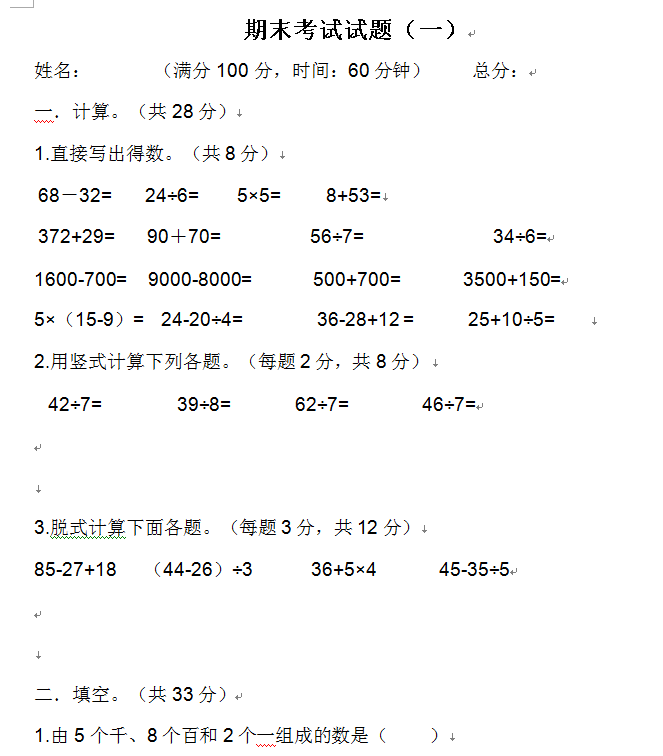1.直接写出得数。（共8分）
68－32=      24÷6=       5×5=        8+53=
372+29=      90＋70=            56÷7=              34÷6=

1600-700=    9000-8000=       500+700=       3500+150=

5×（15-9）=    24-20÷4=          36-28+12 =      25+10÷5=
2.用竖式计算下列各题。（每题2分，共8分）
42÷7=              39÷8=            62÷7=        46÷7=##### 评论信息